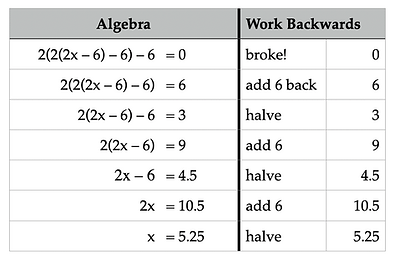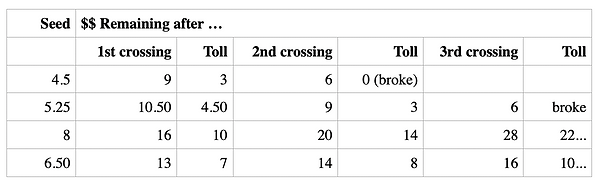top of page

Math Journeys, Volume 3

Boy, Devil, Fractal

Part 1

This journey will show that a rich problem can provide much learning over quite a range of mathematical sophistication. "The Boy and the Devil" can be solved using simple arithmetic or basic algebra, and, by asking questions upon reflection, it can be used to introduce the topics of function, recursion and even escape-time fractals.The problem involves a boy with some money, the Devil, and a bridge. The Devil offers the boy a financial enticement. He will double the youth's holdings every time he crosses the bridge. There will be a modest six-dollar toll to pay at the far end. After one crossing, the boy, in fact, has his money doubled and then he pays the toll. Thinking that he must get rich in the long run, the boy crosses twice more under these rules and finds himself flat broke! How much money did he start with?This journey doesn't begin with a brisk sleigh ride, does it? It's rather more like a slog through a swamp. Where's the trail map? ... the information booth? We don't see many numbers. To solve we must make good use of what little there is to go on. Hmm. Doubled. Pays the \$6 toll. In that order. Oh yes, and 'flat broke' relates to a number.

Solvers have been observed using the following strategies to solve the problem: trial and error, working backwards, writing formulas, and solving an equation.

Trial and Error: Let's have the boy start with \$8. After a crossing he has \$16 and pays \$6, leaving him with \$10. Continuing for two more crossings he ends up with \$22. Too bad he didn't start with \$8. Your assignment is to pick 10 other starting amounts and calculate the results after three or more crossings. Do all 10 trials because we may find that the incorrect answers hold information as well.

Working Backwards: You must use inverse operations in reverse order. If the lad ended with zero dollars, he must have had 0 + 6 before he paid the last toll, and he must have had \$3 before the final doubling. In order to work backwards you must add 6 and halve the amount for each crossing. How much would he have started with before the second crossing to wind up with \$3 left after doubling and paying the toll? Go ahead and carry this through to the solution. You likely will be more efficient than when guessing, but you will know one result and not the 10 from the trials above.

Formulas: If we let x stand for the starting amount, the sequence of three crossings can be modeled by
2x – 6 = y

2y – 6 = z

2z – 6 = 0

Algebra: Hmm. That's quite a few variables. We can eliminate some of them. We have a z in the third formula, but the second formula tells what z is equal to, and so we substitute for it.

2(2y – 6) – 6 = 0

The first formula gives us a replacement for y. We end up with a formula that contains one variable, and it stands for the solution.

2(2(2x – 6) – 6) – 6 = 0

Looks a little busy, eh? But look closely. The inner set of parentheses shows what's in the boy's pocket after one crossing. Likewise, the outer set shows the pocket contents after two crossings. Wow! Parentheses and pockets! To solve this latest equation you perform exactly the steps you used to work the problem backwards.

Compare...Reflection: Our Boy started with \$5.25. Let's mess with this problem. What's so special about a \$6 toll? Would the answer be any different if the toll were, say, \$0.32 or \$8? Solve for the starting amount using these tolls, and try one other toll of your choosing. Look for a connection between solutions and tolls.

In your 10 trials, you probably saw the boy go broke in fewer than three crossings. Solve this question for starting amounts: What if the boy crosses 2, 4 or 5 times before going flat broke paying the last \$6 toll? Look for connections or patterns.

If you think of other ways to mess with Boy & Devil, try them and let me know your results. I've got one more way: Solve the original problem after replacing the word “doubling” with the word "tripling." Use \$0.27 and \$5.40 for tolls.

In part 2, we'll compare our results and make more messes in general.Part 2

We have begun a math journey by solving one problem repeated here:

A boy meets a devil at the entrance to a bridge. The devil determines that the boy has some money, and offers to double the boy's money each time he crosses the bridge, as long as the boy pays the \$6 toll at the far end. On first crossing the boy's money doubles and he gladly pays the toll, but after three crossings the boy is flat broke. How much did he start with?

The answer, \$5.25, was found using three different strategies: trial and error, working backwards, and solving an equation. I'll demonstrate each method by solving for different bridge tolls under the same rules.

Trial and error: What happens if the toll is 32 cents and the boy starts with 25 cents?

Double 25 is 50, less 32 is 18; double 18 is 36, less 32 is 4 cents; double 4 is 8, but the boy does not have the third toll. The list that tracks these amounts is called an orbit, and for this example the orbit in cents is 25, 50, 18, 36, 4, and ... -28, if you will.

In your Part 1 trials did you discover about starting amounts that would allow the boy to gain on the devil? Try one example for yourself. Our trial shows that he must've begun with more than 25 cents. Let's put 30 cents in the boy's pocket. The orbit will be 30, 60, 28, 56, 24, 48, 16, 32, and 0. This orbit shows the boy crossing 4 times, but if you look from 28 cents onward, you see that 28 is the exact amount that allows 3 crossings. So these trials, whether involving the solution or not, possess information. If you have trials from the original problem in Part 1 (10 different starting amounts for a \$6 toll), then save them for Part 3 of this journey.

Working backwards: To work backwards from zero, we add back the toll and halve. If the toll were \$8 and the boy crossed three times, solve for the starting amount.

Solving an Equation: Let's solve for a third different toll, that of \$16. The equation uses a nest of parentheses to show the boy's pocket contents after each crossing. For a toll of \$16, the equation is

2(2(2x – 16) – 16) –16 = 0

Can you solve it?

Looking for a Pattern: Let's put all the results in a table.

Is there a relationship between the starting amount and the toll? It seems that in every case the starting amount is 7/8 of the toll. This general solution can be verified by replacing 16 with T in the equation above and following the same solving steps.

Flexing the problem: Using 32 cents as the toll and varying the number of bridge crossings can be revealing. For one crossing only, The boy would begin with 16 cents, have it doubled, and pay the toll. He would have started with 1/2 the toll. For 2, 3, 4, and 5 crossings, solve for the starting amounts and the connections to the 32-cent toll.

Tripling Question from Part 1: If we flex the problem to investigate "tripling" rather than "doubling" will powers of three be in evidence? Try \$0.27 and \$5.40 for tolls and see.

In part 3 we take this problem up a level by analyzing these orbits, and in the process, we get an understanding of the concepts involved in escape-time fractals.

To see other orbit examples we included some Orbit Posers (pdf) for you.Part 3Uncle Bob now attempts to demonstrate a connection between the arithmetic we've been using to analyze the Boy and the Devil problem, and the colorful and varied graphs commonly called fractals.

The one in the illustration at the left is a prominent example known as the Mandelbrot set, the M-set.

Let observe some of the M-set's qualities. It is two-dimensional. We see it has black regions surrounded by a lacy border. This border separates the black interior from the banded, many-colored exterior.

Now let's revisit the original Boy and Devil problem. See Parts 1 and 2. You may have tried different starting amounts to see what possible fates awaited a boy who continues to play this game. The trails of intermediate amounts of money possessed by the Boy are known as orbits. Here are some examples.The fate of various starting amounts can be mapped on a number line. The various seeds fall into bands of color that distinguish how rapidly or slowly the orbits escape (cool colors) or go broke (warm colors). In between the attractor zero and the fixed point \$6 I've indicated amounts that allow the boy to cross the bridge once, twice or three times.

Fractals are graphs that keep track of orbits too. These orbits result from repetitions of a rule not unlike the Devil's rule applied to various seeds. The graph interiors, usually black, display the regions of stability, in other words, seeds that don't escape. In those regions there may be attractor points. The exterior regions are arbitrarily colored to show the ease with which seeds escape under the rule.

One difference in fractal graphs is that the seeds and the rules apply to complex numbers and are mapped onto the complex plane. Complex numbers have a real part and an imaginary one. This explains why the graphs need to be two-dimensional. The horizontal scale is the same real line we used to map the boy's outcomes. The vertical axis is scaled in multiples of the imaginary number i. To do fractal analysis one needs to understand arithmetic with these complex numbers under repetitions of a rule, and how to sort and graph the seeds. Despite its name, complex arithmetic is not that complicated. You can get a start on understanding it here.

A second difference in fractals is that the boundary is infinitely complex. Zooming in on a portion of the boundary leads to more and more complexity similar to the original portion. These zooms can be seen on the internet. One with a musical rendering of the story can be viewed here.

Mathematicians and scientists are now using fractal math to analyze the complex systems in nature such as watersheds and forest growth on a large scale, and circulatory and nervous systems on a minute scale.

Questions for you. What if the Devil promised to square the amount of money each time the boy crossed? You can create a sampling of orbits of real seeds under repeated squaring. Are there non-escaping values? ... a boundary? ... fixed points? ... an attractors?

To help answer the questions, here is a table of orbits of real numbers under iteration of the squaring function.The seed one (1) is a fixed point and it is a boundary between escaping and non-escaping orbits. Zero is an attractor for seeds between -1 and +1. Any seed with an absolute value greater than one escapes to positive infinity sooner or later.